Caso de estudo-DepreciaçãoPara uma experiência de aprendizado mais agradável, recomendamos que você estude a versão republicada amigável deste curso.

- or -

Continue studying this course

# Caso de estudo-Depreciação

• Study Reminders

We'll email you at these times to remind you to study

You can set up to 7 reminders per week

#### You're all set

We'll email you at these times to remind you to study

Monday

Tuesday

Wednesday

Thursday

Friday

Saturday

Sunday

• Nota de Estudos
• Rever Tópicos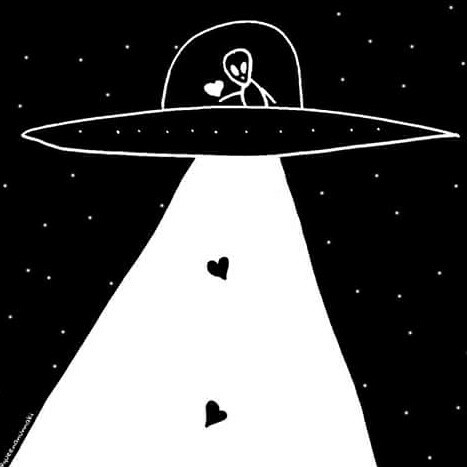Tashika B. 0 0 The case study is very interesting.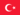BabaJide Martins F. 0 0 The accumulated depreciation represents the amount of the cost of an asset allocated as an expense added up over a number of accounting periods.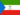Alice B. 0 0 Please can some one tell me if there are two ways of calculating depreciation under the straight line method because on previous topic an example was given and after using this method I got the result of the diminishing balance.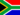Morne V. 0 0 information about diminishing and straight line depreciation method through illustration is clearly given.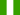Sunday O. 0 0 Case study - Depreciation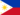Roselyn S. 0 0 Thank you it helps to my further understanding..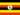Odongo M. 0 0 Accounting -> Case study - Depreciation Case study - Depreciation Example A Pizza Oven is bought on 30 September 2001 for \$10 000. It costs a further \$5000 to install. The residual value is estimated at \$3000 and the estimated life is 6 years. Required: calculate depreciation for the year ended 30.6.2002 and 30.6.2003 using both the straight line method and the diminishing balance (25% per annum) present these calculations in the general journal show the affect on the Profit and Loss statement (extract provided) show how an extract may appear in the balance sheet Straight line Profit and Loss for year ended 30.6.2002 \$ \$ Sales 8 000 less Expenses 4 000 + depreciation of pizza oven Total expenses Net profit Straight line Profit and Loss for year ended 30.6.2003 \$ \$ Sales 8 000 less Expenses 4 000 + depreciation of pizza oven Total expenses Net profit Diminishing balance Profit and Loss for year ended 30.6.2002 \$ \$ Sales 8 000 less Expenses 4 000 + depreciation of pizza oven Total expenses Net profit Diminishing balance Profit and Loss for year ended 30.6.2003 \$ \$ Sales 8 000 less Expenses 4 000 + depreciation of pizza oven Total expenses Net profit A related theory question would ask students to explain the relative effects on profit of the selection of the depreciation method. View the word document for solution to Case study - DepreciationSaul K. 0 0 great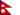Manish K. 0 0 information about diminishing and straight line depreciation method through illustration is clearly given.Davidson Ade O. 0 0 understood### Você recebeu uma nova notificação

Clique aqui para visualizar todos eles